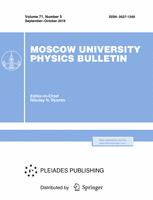Faculty of Physics
M.V.Lomonosov Moscow State University

# Modeling of electron irradiation treatment on kinetics of bacterial content in minced fish

## A. P. Chernyeav$^{1,2}$, V. M. Avdyukhina$^1$, U. A. Bliznyuk$^1$, P. U. Borchegovskaya$^1$, I. C. Gordonova$^3$, V. S. Ipatova$^1$, V. A. Leontyev$^1$, Z. K. Nikitina$^3$, V. V. Rozanov$^1$, F. R. Studenikin$^1$, D. S. Yurov$^2$

### Memoirs of the Faculty of Physics 2020. N 2.

• Article
Annotation

This study illustrates the mathematical modeling of the experimental study on the impact of 1 MeV electrons at different doses on the concentration of bacteria in the homogenate of minced trout during 15 days of storage at a temperature of (2-4)◦C. A mathematical model was constructed based on the general predator-prey model, which describes the change in the number of two species of bacterial populations under conditions of a limited nutrient resource over time. This model agrees well with the experimentally obtained dependences of the change in the concentration of viable cells in minced fish homogenate after exposure to accelerated electrons in various doses.

Approved: 2020 June 29
PACS:
87.53.-j Effects of ionizing radiation on biological systems
Rissian citation:
А. П. Черняев, В. М. Авдюхина, У. А. Близнюк, П. Ю. Борщеговская, И. К. Гордонова, В. С. Ипатова, В. А. Леонтьев, З. К. Никитина, В. В. Розанов, Ф. Р. Студеникин, Д. С. Юров.
Учен. зап. физ. фак-та Моск. ун-та. 2020. № 2. 2020401.
Authors
A. P. Chernyeav$^{1,2}$, V. M. Avdyukhina$^1$, U. A. Bliznyuk$^1$, P. U. Borchegovskaya$^1$, I. C. Gordonova$^3$, V. S. Ipatova$^1$, V. A. Leontyev$^1$, Z. K. Nikitina$^3$, V. V. Rozanov$^1$, F. R. Studenikin$^1$, D. S. Yurov$^2$
$^1$Faculty of Physics M.V.Lomonosov Moscow State University
$^2$Lomonosov Moscow State University, Skobeltsyn Institute of Nuclear Physics, Moskow 119991, Russia.
$^3$All-Russian Scientific Research Institute of Medicinal and Aromatic Plants### Moscow University Physics Bulletin### Science News of the Faculty of Physics, Lomonosov Moscow State University

This new information publication, which is intended to convey to the staff, students and graduate students, faculty colleagues and partners of the main achievements of scientists and scientific information on the events in the life of university physicists.